#Function Repository Resource:

# CircleFit

Find the best-fit circle for a set of points

Contributed by: Sander Huisman
 ResourceFunction["CircleFit"][pts] returns the best-fit circle for the points pts. ResourceFunction["CircleFit"][w→pts] returns the best-fit circle for the points pts having the weights w. ResourceFunction["CircleFit"][w→pts,"Association"] returns an association with the center, the radius, the Circle, a pure function etc.

## Details

ResourceFunction["CircleFit"] returns a Circle object or an Association.
For 1 point, a circle at that point with 0 radius is returned.
For 2 points, a circle at the midpoint with a radius equal to half the distance between the points is returned.
For 3 points that are not collinear, an exact solution will be returned.
For 4 or more points pts, ResourceFunction["CircleFit"] returns the circle centered at {x,y} with radius r that minimizes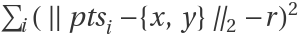.
For 4 or more points pts and weights w, ResourceFunction["CircleFit"] returns the circle centered at {x,y} with radius r that minimizes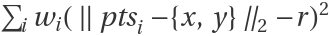.

## Examples

### Basic Examples (2)

Find the circle through 4 points and visualize the result:

 In:=Out=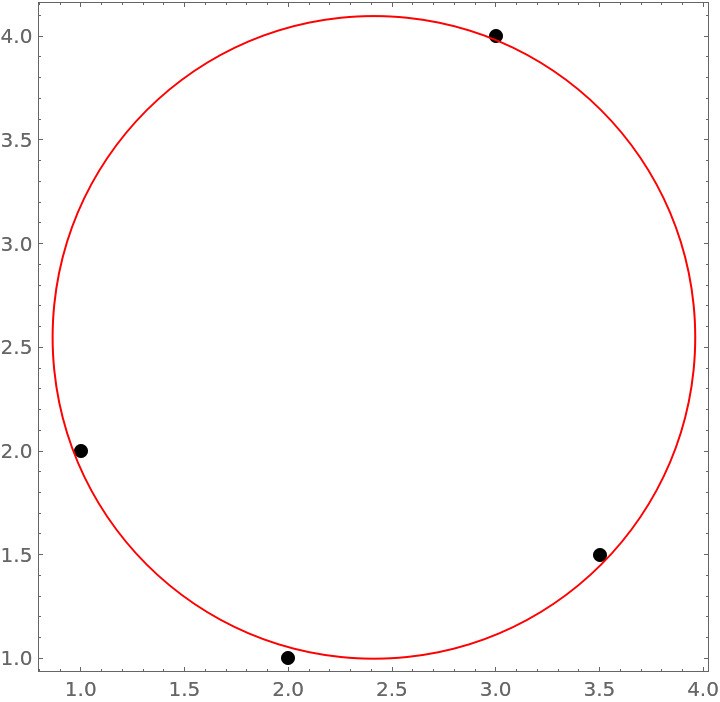Find the best-fitting circle for a 2500 points:

 In:=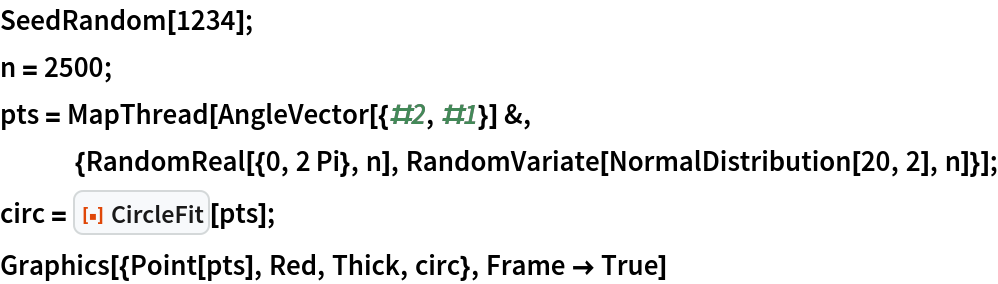Out=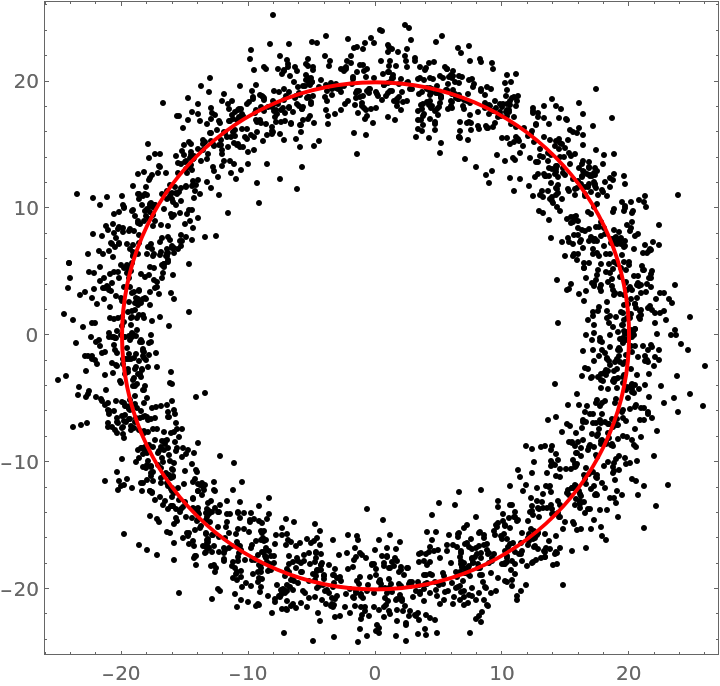### Scope (2)

Specify weights:

 In:=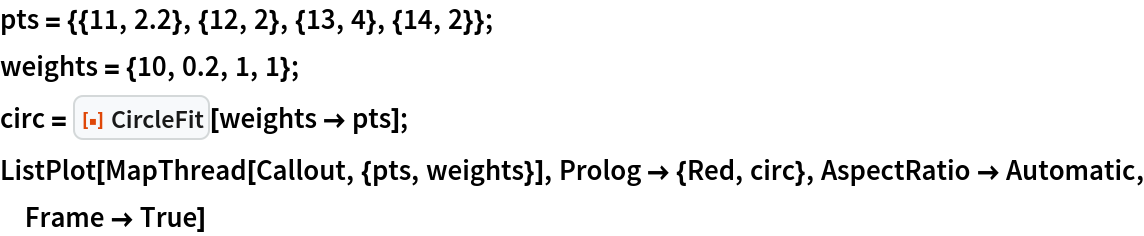Out=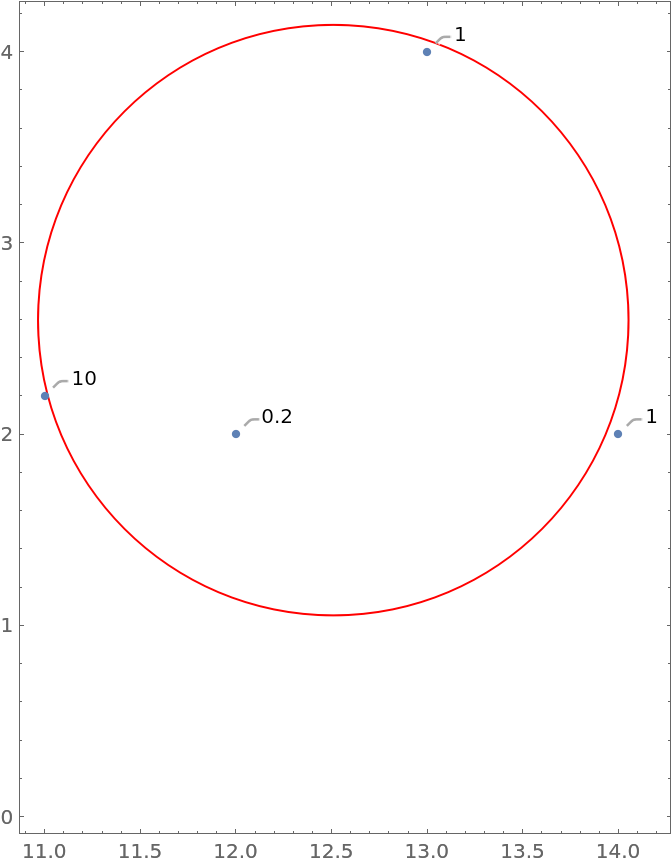Return an Association with all the details:

 In:=Out=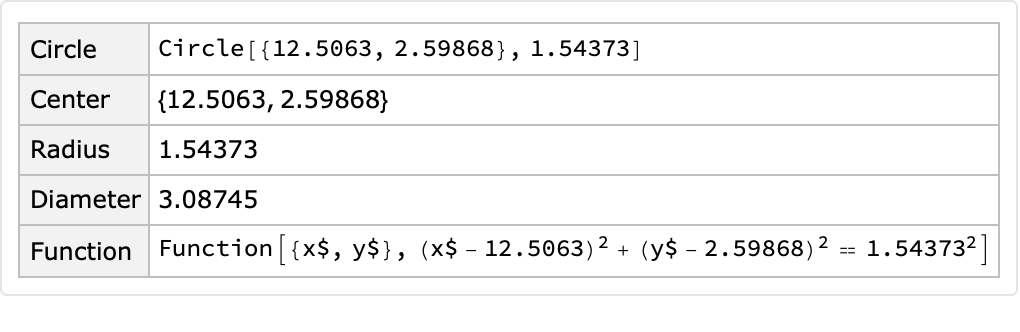### Properties and Relations (1)

For 2 points, the center of the circle is the midpoints of the points:

 In:=Out=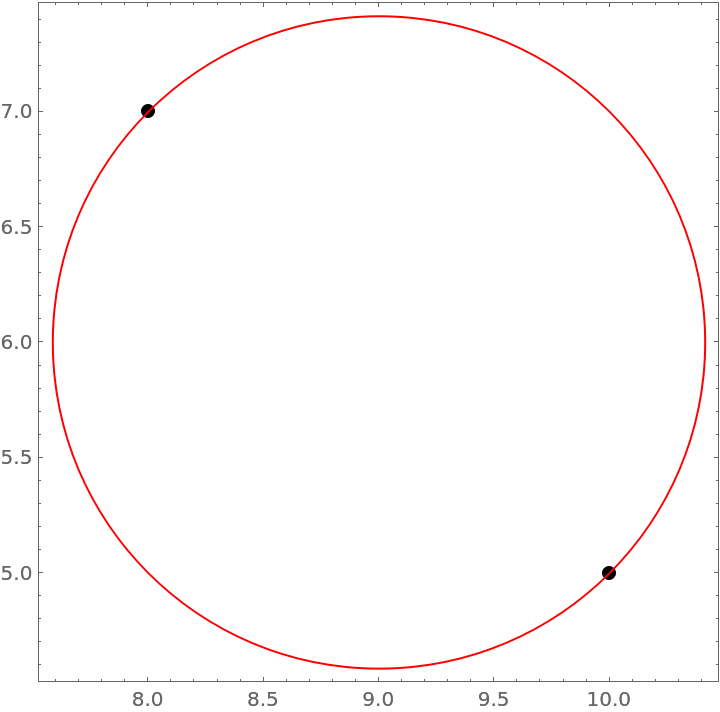### Possible Issues (3)

When no points are given, a Failure object is returned:

 In:=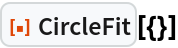Out=When 3 points are given and they are collinear, a Failure object is returned:

 In:=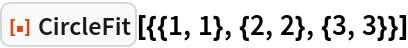Out=The case of multiple collinear points results in circle that is far away with a large radius:

 In:=Out=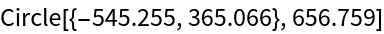### Neat Examples (1)

Find the best-fitting circle for points clustered around a circular arc:

 In:=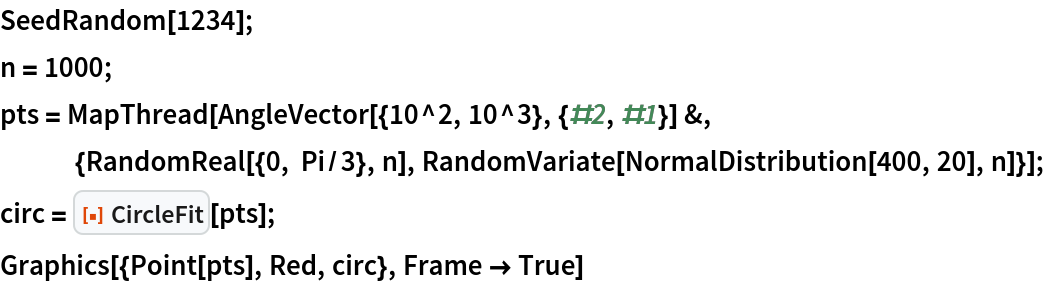Out=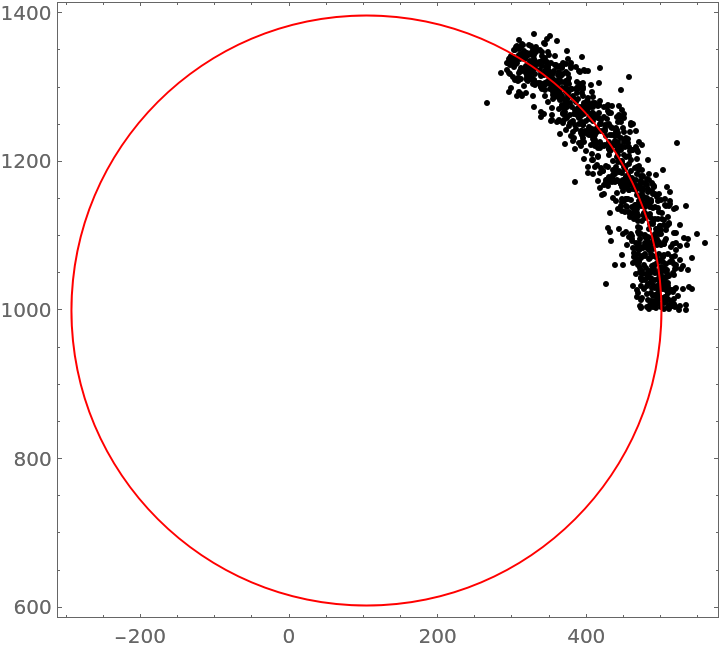## Resource History

First an estimate is found by minimizing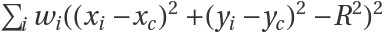. After that the real problem is minimized using the estimate as a starting points,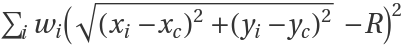.